• 输入任意的上下文无关文法,输出所输入的上下文无关文法一切非终结符的first集合和follow集合
• C语音代码。实现功能：1....2.求每个非终结符FIRST FOLLOW和SELECT模块。3.预测分析表的构建模块。4.文法的检验及消除左公因子和左递归模块。5.对输入终结符串的判断，是否为LL1文法，并进一步分析。select
• 代码链接：https://pan.baidu.com/s/1VNdrSMXaKu3HI0UQ_TInUQ 提取码：b1qz 使用教程 解压后打开文件夹，直接用Dev c++运行LL1，如图： 即可实现。 一分钱都不要啊， 比那些要C币的都好，点个赞呗亲们！！！ ...

DEV C++ 项目实现 不会建项目的看这个——>如何创建项目

提取码：b1qz

使用教程

解压后打开文件夹，直接用Dev c++运行LL1，如图：即可实现。

一分钱都不要呀， 比需要C币下载的资源都好，点个赞呗！

展开全文编译原理 first集 follow集 代码
• 编译原理之NULLfirst、followC语言实现，实现中句子的转换符号由‘#’代替，数组默认由‘*’作为结束符
• 最近在学习微机原理，里面涉及到编译优化的问题，又去重新看了看龙书的语法分析部分。之前学习的时候只是知道first和follow集合怎么计算，但是没有很明白背后的原理。想起轮子哥的一句话：要理解一个东西最好的办法...

前言

最近在学习微机原理，里面涉及到编译优化的问题，又去重新看了看龙书的语法分析部分。之前学习的时候只是知道first和follow集合怎么计算，但是没有很明白背后的原理。想起轮子哥的一句话：要理解一个东西最好的办法就是实现它。所以就心血来潮，写了C++的实现

代码

思路什么的就不说了，都写在代码的注释里面。

#include<string>
#include<iostream>
#include<vector>
#include <map>
#include <set>
#include <regex>
using namespace std;
const int MAX_N = 5000;
//记录总数
int cnt = 0;
pair<string,vector<string> > exps[MAX_N];
set<string> Vns,Vts;//Vns表示非终结符的集合，Vts表示终结符的集合
//使用set的好处就是可以去掉手工判断重复元素
map<string,set<string> > first;//计算得到的first集合，同时也包含终结符的（也就是它本身。为了方便起见，往这里加入了）
map<string,set<string> > follow;//计算得到的follow集合

//判断是否属于终结符
bool is_terminal_char(const string & s){
//只要不是大写的我们就认为它属于终结符
regex nt("[A-Z]*");
if(regex_match(s,nt)){
return false;
}
return true;
}

string str;
cout<<"input information (end with #):\n";

while (getline(cin,str)){
if(str.find("#") != -1) break;//结束的条件，如果是#就直接跳出循环
//输入的表达式以 -> 作为左右方向的分隔符。所以直接去找这个->的位置
int mid_pos = str.find("->");
if(mid_pos == -1){
cout<<"输入的表达式格式有误，已忽略该表达式\n";
continue;
}
int ls = 0,le = mid_pos;//表示左边的字符串起始位置和结束位置
while (str[ls] == ' ') ls++;

for(int i = ls;i<mid_pos;i++){
if(str[i] == ' '){
le = i;
break;
}
}
//确定表达式左边的非终结符的具体内容
exps[cnt].first = str.substr(ls,le-ls);
Vns.insert(exps[cnt].first);//加入到终结符的集合中
//cout<<"--"<<exps[cnt].first<<"--"<<endl;
//继续往后读取，把表达式右边的字符全都加入进来
for(int i = mid_pos + 2; i<(int)str.length();i++){
//表达式右边把所有的单字符都读入到vector里面
if(str[i] == ' ') continue;
exps[cnt].second.push_back(str.substr(i,1));
string exp_elem = str.substr(i,1);
if(is_terminal_char(exp_elem)){
Vts.insert(exp_elem);
first[exp_elem].insert(exp_elem);
}
}
//读取完毕后，把cnt+1
cnt++;
//        for(string s : exps[cnt].second){ // 读取测试完成
//            cout<<s<<" ";
//        }
}
}

//计算first集合
void cal_first(){
int pre_size = -1,now_size = 0;
//设置更新的条件
while(pre_size != now_size){
pre_size = now_size;
for(int i = 0;i<cnt;i++){
string str = exps[i].first;
vector<string> elements = exps[i].second;
//如果说表达式右边的第一个字符属于终结符，那么就直接把它加入到first(str)中(规则1)
if(is_terminal_char(elements)){
first[str].insert(elements);
}
//规则2
else {
for (int j = 0; j < (int) elements.size(); j++) {
if (is_terminal_char(elements[j])) {//用于循环体判断。如果发现已经到达了终结符的位置，就退出
break;
}
//将两个集合进行合并
for(string tmp : first[elements[j]]){
//注意，空字符不能够加入到str的first集合中
if(tmp != "~"){
first[str].insert(tmp);
}
//但是如果发现所有的非终结符都可能推导出空字符，那么就把空字符加进去
else if(j == elements.size() - 1){
first[str].insert(tmp);
}
}
//如果发现没有空字符集，就直接退出去
if (first[elements[j]].find("~") == first[elements[j]].end()) {
break;
}
}
}
now_size = 0;
for(string tmp : Vns){//重新计算now_size的大小
now_size += (int)first[tmp].size();
}
}
}
}

void cal_follow(){
//使用规则1
follow["S"].insert("#");//默认S为文法的起始字符
//这两个标志只用于判断是否已经生成了完全的follow集合
int pre_size = -1,now_size = 0;
while (pre_size !=  now_size){
pre_size = now_size;
for(int i = 0;i<cnt;i++){
string str = exps[i].first;
vector<string> elements = exps[i].second;
//使用规则2
for(int j = 0;j<(int)elements.size()-1;j++){
if(!is_terminal_char(elements[j])){//如果当前的是非终结符
//并且后面的那个单元也是非终结符
//就把后面的那个非终结符除~以外的所有first元素都加入进去
follow[elements[j]].insert(first[elements[j + 1]].begin(), first[elements[j + 1]].end());
//去掉空字符
follow[elements[j]].erase("~");
//否则就把后面的单个终结符加入进去
}
}
//如果当前的是终结符，那么规则2就不用了.
//使用规则3,为了方便起见，从后往向前遍历
for(int j = elements.size() - 1;j>=0;j--){
//如果是非终结符，就把推导式中的follow元素都加入到它对应的follow集合里面
if(!is_terminal_char(elements[j])){
follow[elements[j]].insert(follow[str].begin(),follow[str].end());
}
else break;//如果是终结符，就直接退出

//表明在first集合中没有空字符，这样的话就直接退出
if(first[elements[j]].find("~") == first[elements[j]].end()){
break;
}
}
}
now_size = 0;
for(string tmp : Vns){//重新计算now_size的大小
now_size += (int)follow[tmp].size();
}
}
}

/**
* testData is in test_data.txt
* @return
*/
int main(){
Vts.insert("#");

cal_first();
cout<<"---first---\n";
for(string n : Vns){
cout<<n<<":";
for(string tmp : first[n]){
cout<<tmp<<" ";
}
cout<<endl;
}
cal_follow();
cout<<"---follow---\n";
for(string n : Vns){
cout<<n<<":";
for(string tmp : follow[n]){
cout<<tmp<<" ";
}
cout<<endl;
}
return 0;
}

测试数据：
输入：

S->AB
S->bC
A->~
A->b
B->~
C->b
D->aS
D->c

预计输出:

---first---
A:b ~
B:a ~
C:a b c
D:a c
S:a b ~
---follow---
A:# a c
B:#
C:#
D:#
S:#

当然设计的还有很多不完善的地方，后续自己优化吧。

展开全文编译器 算法 c++ stl
• 下面是这部分的代码，我贴一部分，剩下的等我把求follow实现再写一篇博文来给大家看！ 请给我点赞谢谢~！！！ 实现得非常简洁明了（自夸） 你可以看下注释，写的非常清楚啦!!! package parse2; import java.u...

哈哈，经历了千辛万苦，我！终于！写出来了！
其实总体说来也不难，但是我比较傻，想来想去都想不通，现在终于写出来了，请大家分享一下我的快乐~~~~~~哈哈哈！！
下面是这部分的代码，我贴一部分，剩下的等我把求follow集实现再写一篇博文来给大家看！
请给我点赞谢谢~！！！
实现得非常简洁明了（自夸）
你可以看下注释，写的非常清楚啦!!!

package parse2;

import java.util.ArrayList;
import java.util.Iterator;
import java.util.List;
import parse2.Production;

List<Production> proList = new ArrayList<Production>();

public FirstFollow(ProductionList productionList) {
proList = productionList.getProductions();
}

/**
* 判断是不是终结符，如果左边没这个作为开头的，那就是终结符了。
* @param str
* @return
*/
public boolean isVariable(String str) {
for (Iterator<Production> iterator = proList.iterator(); iterator
.hasNext();) {
Production production = (Production) iterator.next();
if (production.getLeft().equals(str)) {
// 一旦找到左边有等于str的字符，就说明str不算终结符，返回真：是变量
return true;
}
}
return false;
}

/**
* 判断是不是空产生式集
* @param str
* @return
*/
public boolean isEmpty(String str) {
for (Iterator<Production> iterator = proList.iterator(); iterator
.hasNext();) {
Production production = (Production) iterator.next();
if (production.getLeft().equals(str)) {
for (int i = 0; i < production.getRight().length; i++) {
// System.out.println(production.getRight()[i]);
if (production.getRight()[i].equals("null")) {
return true;
}
}
}
}
return false;
}

/**
* 返回包含这个左部的产生式集合，
* @param B
* @param productions
* @return
*/
public List<Production> findLeft(String B) {
List<Production> list = new ArrayList<>();
for (Iterator<Production> iterator = proList.iterator(); iterator
.hasNext();) {
Production production = (Production) iterator.next();
// System.out.println(production.getLeft());
if (production.getLeft().equals(B)) {
}
}
return list;
}

/**
* 获取非终结符号的产生式的first集合X->Y1Y2Y3Y4Y5……这样的,
* @param str X
* @return
*/
public List<String> getFirstItem(Production production) {
List<String> list = new ArrayList<>();// 获取包含这个str左部的产生式
// 遍历这个产生式的每一项，其中每个产生式的每一项也需要遍历。
for (int i = 0; i < production.getRight().length; i++) {
if (!production.getLeft().equals(production.getRight()[i])) {
System.out.println(production.getRight()[i]);
} // 没有左递归
if (!isEmpty(production.getRight()[i])) {
// 这个项里没有包含空产生式的话，就继续求解，否则结束。
return list;
}

}
/* List<Production> findList = findLeft(str);

for (Iterator<Production> iterator = findList.iterator(); iterator
.hasNext();) {
Production production = (Production) iterator.next();
for (int i = 0; i < production.getRight().length; i++) {
System.out.println(production.getRight()[i]);
if (!isEmpty(production.getRight()[i])) {
return list;
}
}
}*/
return list;
}

/**
* 判断是不是空产生式集
* @param strings
* @return
*/
public boolean isEmpty(String[] strings) {
for (int i = 0; i < strings.length; i++) {
if (strings[i].equals("null")) {
return true;
}
}
return false;
}

/**
* 获取first集合
* @param str
* @return
*/
public List<String> getFirst(String str) {
List<String> list = new ArrayList<>();
List<Production> productions = findLeft(str);
System.out.println(productions);
for (Iterator<Production> iterator = productions.iterator(); iterator
.hasNext();) {
Production production = (Production) iterator.next();
if (isEmpty(production.getRight())) {
System.out.println("-------------------null------------------");
// 检查X->null是否成立
} else if (!isVariable(production.getRight())
&& !isEmpty(production.getRight())) {
// 是终结符的话就直接加入。
System.out.println("-------------------vict------------------");
} else {
System.out.println("-------------------set------------------");
}
}
return list;
}

public static void main(String[] args) {
ProductionList productionSet = new ProductionList();
System.out.println(productionSet.toString());
System.out.println(firstFollow.getFirst("T'"));;
}
}

效果示意:
我用的产生式如下:
龙书（紫色第二版）P140的例子文法，当然其他的文法也不在话下~~
另外注意，左递归不消除的话可能会导致循环哦！！！

E -> T E'
E' -> + T E'|null
T -> F T'
T' -> * F T'|null
F -> ( E )|id

代码运行结果：这里的null表示空~！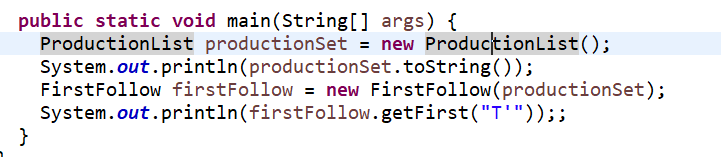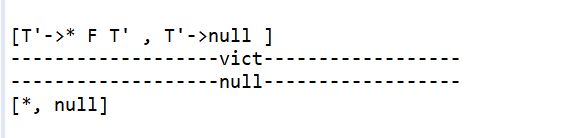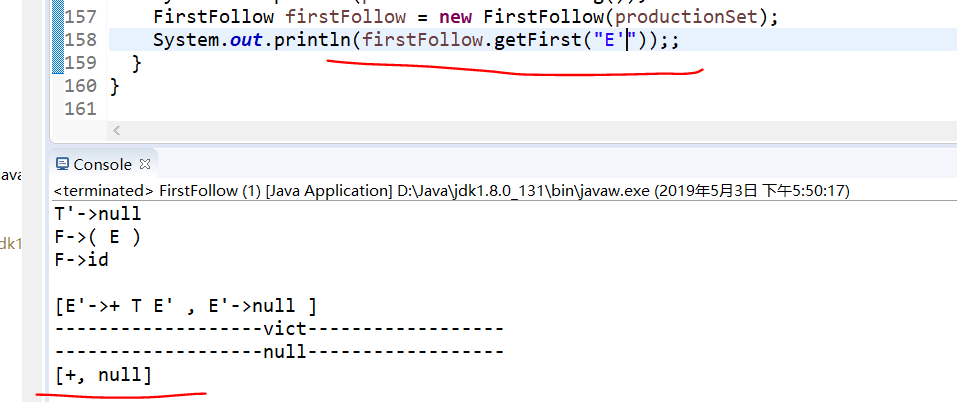现在的心情非常激动~~！

展开全文编译原理 java
• ( 编译原理JAVA求First集Follow
• 语言为C++，使用了set，map容器，输入格式：S -> Aa | g | e，支持多‘|’ 符号，采用文件输入
• 编译原理实验 first、follow、select集合的求解，经测试正确，c语言编写first follow
• 文章目录FIRST集的求法FOLLOW的计算生成预期分析表算法例题 FIRST集的求法 FIRST集是一个文法符号串所可能推导出的符号串的第一个终结符的集合 对于文法G的任一符号串α\alphaα = x1x2…xnx_{1}x_{2} \ldots x_{n...

FIRST集的求法

FIRST集是一个文法符号串所可能推导出的符号串的第一个终结符的集合
对于文法G的任一符号串 α \alpha = x 1 x 2 … x n x_{1}x_{2} \ldots x_{n}

1. 置 FIRST( α \alpha ) = ϕ \phi
2. 将 FIRST( x 1 x_{1} ) 中一切非 ϵ \epsilon 符号加进 FIRST( α \alpha )
3. ϵ ∈ \epsilon\in FIRST( x 1 x_{1} ), 将 FIRST( x 2 x_{2} ) 中一切非 ϵ \epsilon 符号加进FIRST( α \alpha ); 若 ϵ ∈ \epsilon \in FIRST( x 1 x_{1} ) 和 FIRST( x 2 x_{2} ), 将 FIRST( x 3 x_{3} ) 中一切非 ϵ \epsilon 符号加进 FIRST( α \alpha ) 中, 依此类推.
4. 对于一切 1 ⩽ \leqslant i ⩽ \leqslant n, ϵ ∈ \epsilon \in FIRST( x i x_{i} ), 则将 ϵ \epsilon 符号加入 FIRST( α \alpha ).

note:
FIRST集从产生式左侧推导，例如求A的FIRST集，要先从产生式左侧找到A，然后根据产生式右侧的信息求出A的FIRST集

例如：
S → \rightarrow BS ‘ ^
S ‘ → ^ \rightarrow aB | ϵ \epsilon
B → \rightarrow DB ‘ ^
B ‘ → ^ \rightarrow b | ϵ \epsilon
D → \rightarrow d | ϵ \epsilon

FIRST(S) = {d, b, a, ϵ \epsilon }
FIRST(S ‘ ^ ) = {a, ϵ \epsilon }
FIRST(B) = {d, b, ϵ \epsilon }
FIRST(B ‘ ^ ) = {b, ϵ \epsilon }
FIRST(D) = {d, ϵ \epsilon }

解释：
第一个求S的FIRST集，先从产生式的左侧找到S, (S → \rightarrow BS ‘ ^ ), 然后根据该产生式右侧的信息(BS ‘ ^ ) 和求FIRST集的第二条规则求FIRST(B), 从产生式的左侧找到B, (B → \rightarrow DB ‘ ^ ), 然后根据该产生式右侧的信息(DB ‘ ^ ) 和求FIRST集的第二条规则求FIRST(D), 从产生式的左侧找到D, (D → \rightarrow d | ϵ \epsilon ), 然后根据该产生式右侧的信息(d | ϵ \epsilon ) 和求FIRST集的第二条规则, 将终结符d加入FIRST(S).
F I R S T ( S ) = { d } \color{red} { FIRST(S) = \{d\} }
又根据第三条规则，因为D → ϵ \rightarrow \epsilon , 所以将FIRST(B ‘ ^ ) 加入到FIRST(S)中，从产生式的左侧找到B ‘ ^ , (B ‘ → ^ \rightarrow b | ϵ \epsilon ), 然后根据该产生式右侧的信息(b | ϵ \epsilon ) 和求FIRST集的第二条规则, 将终结符b加入FIRST(S).
F I R S T ( S ) = { d , b } \color{red} { FIRST(S) = \{d, b\} }
又根据第三条规则，因为B ‘ → ϵ ^ \rightarrow \epsilon , 所以将FIRST(S ‘ ^ ) 加入到FIRST(S)中，从产生式的左侧找到S ‘ ^ , (S ‘ → ^ \rightarrow aB | ϵ \epsilon ), 然后根据该产生式右侧的信息(aB | ϵ \epsilon ) 和求FIRST集的第二条规则, 将终结符a加入FIRST(S).
F I R S T ( S ) = { d , b , a } \color{red} { FIRST(S) = \{d, b, a\} }
又根据第四条规则，因为 ϵ ∈ \epsilon \in FIRST(D) , ϵ ∈ \epsilon \in FIRST(B ‘ ^ ), 所以 ϵ ∈ \epsilon \in FIRST(B), 又因为 ϵ ∈ \epsilon \in FIRST(S ‘ ^ ), 所以 ϵ ∈ \epsilon \in FIRST(S). 最终结果如下:
F I R S T ( S ) = { d , b , a , ϵ } \color{red} { FIRST(S) = \{d, b, a, \epsilon\} }

第二个求S ‘ ^ 的FIRST集, 先从产生式左侧找到S ‘ ^ , (S ‘ → ^ \rightarrow aB | ϵ \epsilon ), 然后根据产生式右侧的信息(aB | ϵ \epsilon ), S既能推导出aB又能推导出 ϵ \epsilon , 所以根据规则二和规则四得出:
F I R S T ( S ‘ ) = { a , ϵ } \color{red}{ FIRST(S^) = \{a, \epsilon\} }

第三个求B的FIRST集, 产生式的左侧找到B, (B → \rightarrow DB ‘ ^ ), 然后根据该产生式右侧的信息(DB ‘ ^ ) 和求FIRST集的第二条规则求FIRST(D), 从产生式的左侧找到D, (D → \rightarrow d | ϵ \epsilon ), 然后根据该产生式右侧的信息(d | ϵ \epsilon ) 和求FIRST集的第二条规则, 将终结符d加入FIRST(B).
F I R S T ( B ) = { d } \color{red} { FIRST(B) = \{d\} }
又根据第三条规则，因为D → ϵ \rightarrow \epsilon , 所以将FIRST(B ‘ ^ ) 加入到FIRST(B)中，从产生式的左侧找到B ‘ ^ , (B ‘ → ^ \rightarrow b | ϵ \epsilon ), 然后根据该产生式右侧的信息(b | ϵ \epsilon ) 和求FIRST集的第二条规则, 将终结符b加入FIRST(B).
F I R S T ( B ) = { d , b } \color{red} { FIRST(B) = \{d, b\} }
又根据第四条规则，因为 ϵ ∈ \epsilon \in FIRST(D) , ϵ ∈ \epsilon \in FIRST(B ‘ ^ ), 所以 ϵ ∈ \epsilon \in FIRST(B), 最终结果如下:
F I R S T ( B ) = { d , b , ϵ } \color{red} { FIRST(B) = \{d, b, \epsilon\} }

求第四个B ‘ ^ 的FIRST集和第五个求D的FIRST集同求第二个求S ‘ ^ 的FIRST集，结果为:
F I R S T ( B ‘ ) = { b , ϵ } F I R S T ( D ) = { d , ϵ } \color{red} { FIRST(B^) = \{b, \epsilon\} }\\ { FIRST(D) = \{d, \epsilon\} }

FOLLOW集的计算

FOLLOW集是文法符号后面可能跟随的终结符的集合(不包括空串)

1. 对于文法的开始符号S，置 # 于 FOLLOW(S)中.
2. 若 A → \rightarrow aB β \beta 是一个产生式， 则把 FIRST( β \beta ) \ | ϵ \epsilon | 加至 FOLLOW(B) 中.
3. 若 A → \rightarrow aB 是一个产生式，或 A → \rightarrow aB β \beta 是一个产生式而 β ⇒ ϵ \beta \Rightarrow \epsilon (即 ϵ ∈ \epsilon \in ) FIRST(B), 则把 FOLLOW(A) 加至 FOLLOW(B) 中.

note:

1. FOLLOW集中没有 ϵ \epsilon
2. FOLLOW集从产生式右侧推导，例如求A的FOLLOW集时，要从产生式右侧找到A，然后根据A右侧符号信息，求出A的FOLLOW集

例如：
S → \rightarrow BS ‘ ^
S ‘ → ^ \rightarrow aB | ϵ \epsilon
B → \rightarrow DB ‘ ^
B ‘ → ^ \rightarrow b | ϵ \epsilon
D → \rightarrow d | ϵ \epsilon

FOLLOW(S) = {#}
FOLLOW(S ‘ ^ ) = {#}
FOLLOW(B) = FIRST(S ‘ ^ ) \ | ϵ \epsilon | ∪ \cup FOLLOW(S ‘ ^ ) ∪ \cup FOLLOW(S) = {a, #}
FOLLOW(B ‘ ^ ) = FOLLOW(B) = {a, #}
FOLLOW(D) = FIRST(B ‘ ^ ) \ | ϵ \epsilon | ∪ \cup FOLLOW(B) = {b, a, #}

解释：
第一个求S的FOLLOW集, 因为S是开始符号，所以将#加入到FOLLOW(S)中，从产生式右侧找S，没有找到，所以最终结果:
F O L L O W ( S ) = { # } \color{red} { FOLLOW(S) = \{ \# \} }
第二个求S ‘ ^ 的FOLLOW集, 从产生式右侧找S ‘ ^ , (S → \rightarrow BS ‘ ^ ), 根据右侧符号信息和求FOLLOW集的第三条规则，将 FOLLOW(S) 加入到 FOLLOW(S ‘ ^ ) 中，最终结果:
F O L L O W ( S ‘ ) = { # } \color{red} { FOLLOW(S^) = \{ \# \} }
第三个求B的FOLLOW集，从产生式右侧找到B，(S → \rightarrow BS ‘ ^ 、S ‘ → ^ \rightarrow aB | ϵ \epsilon ), 根据 S → \rightarrow BS ‘ ^ 和第二条规则，得:
F O L L O W ( B ) = F I R S T ( S ‘ ) ∖ ∣ ϵ ∣ = { a } \color{red} { FOLLOW(B) = FIRST(S^) \setminus |\epsilon| = \{a\} }
根据S ‘ → ^ \rightarrow aB 和第三条规则，得:
F O L L O W ( B ) = F O L L O W ( S ‘ ) = { a , # } \color{red} { FOLLOW(B) = FOLLOW(S^) = \{a, \# \} }
又因为S ‘ → ϵ ^ \rightarrow \epsilon , 根据S → \rightarrow BS ‘ ^ 和第三条规则，得:
F O L L O W ( B ) = F O L L O W ( S ) = { a , # } \color{red} { FOLLOW(B) = FOLLOW(S) = \{a, \#\} }
第四个求B ‘ ^ 的FOLLOW集，从产生式的右侧找到B ‘ ^ , (B → \rightarrow DB ‘ ^ ), 根据第三条规则，得:
F O L L O W ( B ‘ ) = F O L L O W ( B ) = { a , # } \color{red} { FOLLOW(B^) = FOLLOW(B) = \{a, \#\} }
第五个求D的FOLLOW集，从产生式的右侧找到D, (B → \rightarrow DB ‘ ^ ), 根据第二条规则，得:
F O L L O W ( D ) = F I R S T ( B ‘ ) ∖ ∣ ϵ ∣ = { b } \color{red} { FOLLOW(D) = FIRST(B^) \setminus |\epsilon| = \{b\} }
又因为B ‘ → ϵ ^ \rightarrow \epsilon , 根据 B → \rightarrow DB ‘ ^ 和第三条规则，得:
F O L L O W ( D ) = F O L L O W ( B ) = { b , a , # } \color{red} { FOLLOW(D) = FOLLOW(B) = \{b, a, \#\} }

生成预期分析表算法

1. 对于文法G的每个产生式 A → α \rightarrow \alpha , 执行第2步和第3步
2. 对每个终结符a ∈ \in FIRST( α \alpha ), 把 A → α \rightarrow \alpha 加至 M[A, a]中
3. ϵ ∈ \epsilon \in FIRST( α \alpha ), 则对任何b ∈ \in FOLLOW(A) 把 A → α \rightarrow \alpha 加至 M[A, b]中

note:
每个产生式的意思是将所有含“|”的产生式全部展开，然后看每个产生式的FIRST集，如果这个FIRST集中有“ ϵ \epsilon ”, 那就要将这个产生式加入到 FOLLOW集中相应符号中

例题

已知文法G如下:
S → \rightarrow AB
A → \rightarrow Ba | ϵ \epsilon
B → \rightarrow Db | D
D → \rightarrow d | ϵ \epsilon
(1) 构建文法G的LL(1)分析表

因为 S ⇒ \Rightarrow A ⇒ \Rightarrow B ⇒ \Rightarrow D ⇒ ̸ \Rightarrow \not S, 所以不存在左递归
因为将 A → \rightarrow Ba | ϵ \epsilon 代入到 S → \rightarrow AB 会含有回溯，B → \rightarrow Db | D 这条产生式本身也含有回溯，所以要先消除回溯

消除左递归(本题不需要消除左递归)：
P的产生式: P → \rightarrow Pa 1 _1 | Pa 2 _2 | … | Pa m _m | β 1 \beta_1 | β 2 \beta_2 | … | β n \beta_n
其中，每个a不等于 ϵ \epsilon , 每个 β \beta 不以P开头
P → β 1 P ‘ \rightarrow \beta_1 P^ | β 2 P ‘ \beta_2 P^ | … | β n P ‘ \beta_n P^
P ‘ → a 1 P ‘ ^ \rightarrow a_1 P^ | a 2 P ‘ a_2 P^ | … | a m P ‘ a_m P^

消除回溯(提左因子)
A的规则是: A → δ β 1 \rightarrow \delta \beta_1 | δ β 2 \delta \beta_2 | … | δ β n \delta \beta_n | γ 1 \gamma_1 | γ 2 \gamma_2 | … | γ m \gamma_m
其中，每个 γ \gamma 不以 δ \delta 开头
A → δ A ‘ \rightarrow \delta A^ | γ 1 \gamma_1 | γ 2 \gamma_2 | … | γ m \gamma_m
A ‘ → β 1 ^ \rightarrow \beta_1 | β 2 \beta_2 | … | β n \beta_n

通过消除回溯，将文法改写为:
S → \rightarrow BS ‘ ^
S ‘ → ^ \rightarrow aB | ϵ \epsilon
B → \rightarrow DB ‘ ^
B ‘ → ^ \rightarrow b | ϵ \epsilon
D → \rightarrow d | ϵ \epsilon

通过拆分上边的产生式，得到：

1. S → \rightarrow BS ‘ ^
2. S ‘ → ^ \rightarrow aB
3. S ‘ → ϵ ^ \rightarrow \epsilon
4. B → \rightarrow DB ‘ ^
5. B ‘ → ^ \rightarrow b
6. B ‘ → ϵ ^ \rightarrow \epsilon
7. D → \rightarrow d
8. D → ϵ \rightarrow \epsilon

先看这八个产生式的FIRST集，如果FIRST集中有 ϵ \epsilon , 就要看相应产生式的FOLLOW集(例如产生式3、6、8)

终结符: {a, b, d, #}
非终结符: {S, S ‘ ^ , B, B ‘ ^ , D}

FIRST(S) = {a, b, d, ϵ \epsilon }      FOLLOW(S) = {#}
FIRST(S ‘ ^ ) = {a, ϵ \epsilon }             FOLLOW(S ‘ ^ ) = {#}
FIRST(B) = {d, b, ϵ \epsilon }          FOLLOW(B) = {a, #}
FIRST(B ‘ ^ ) = {b, ϵ \epsilon }             FOLLOW(B ‘ ^ ) = {a, #}
FIRST(D) = {d, ϵ \epsilon }              FOLLOW(D) = {b, a, #}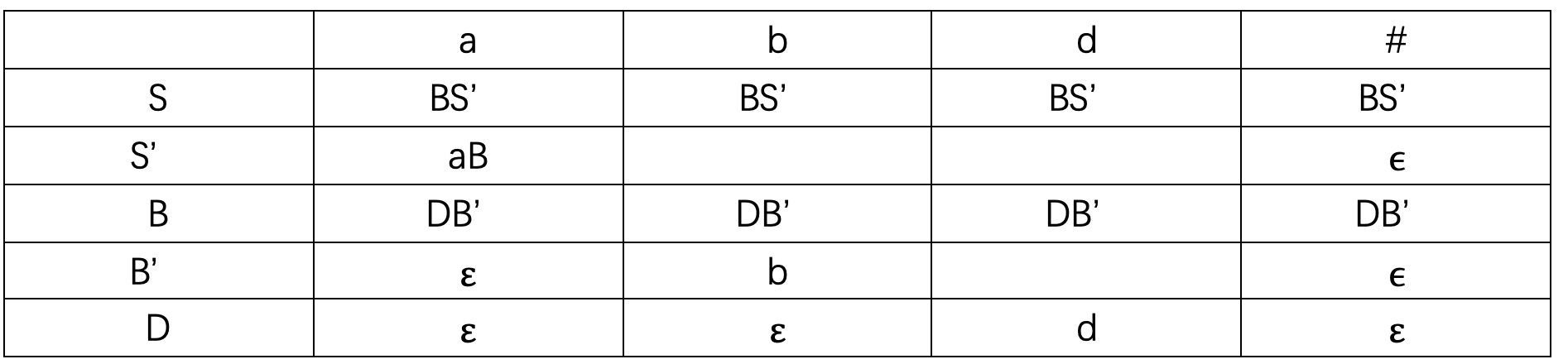解释：
先看S这一行，因为第一条产生式 FIRST(BS ‘ ^ ) = {a, b, d, ϵ \epsilon }，所以根据第二条规则，在a, b, d列填入BS ‘ ^ ，又因为 ϵ ∈ \epsilon \in FIRST(BS ‘ ^ ), 所以还要看S的FOLLOW集，FOLLOW(S) = {#}，所以在#这一列也填入BS ‘ ^
看S ‘ ^ 这一行，第二条产生式 FIRST(aB) = {a}, 根据第二条规则，在a这一列填入aB
又因为第三条产生式S ‘ → ϵ ^ \rightarrow \epsilon , ϵ ∈ \epsilon \in FIRST(S ‘ ^ ), 所以看S ‘ ^ 的FOLLOW集，FOLLOW(S ‘ ^ ) = {#}, 所以在#这一列填入 ϵ \epsilon (这个 ϵ \epsilon 由S ‘ → ϵ ^ \rightarrow \epsilon 这条产生式得出)
看B这一行，因为第四条产生式 FIRST(DB ‘ ^ ) = {d, b, ϵ \epsilon }, 根据第二条规则，在d, b列填入DB ‘ ^ , 又因为 ϵ ∈ \epsilon \in FIRST(DB ‘ ^ ), 所以看B的FOLLOW集，因为FOLLOW(B) = {a, #}, 所以在a, #列填入DB ‘ ^
看B ‘ ^ 这一行，因为第五条产生式 FIRST(b) = {b}, 所以在b列填入b
又因为第六条产生式B ‘ → ϵ ^ \rightarrow \epsilon , ϵ ∈ \epsilon \in FIRST(B ‘ ^ ), 所以看B ‘ ^ 的FOLLOW集，FOLLOW(B ‘ ^ ) = {a, #}, 所以在a, #列填入 ϵ \epsilon
看D这一行，因为第七条产生式 FIRST(d) = {d}, 所以在d列填入d
又因为第八条产生式 D → ϵ \rightarrow \epsilon , ϵ ∈ \epsilon \in FIRST(D), 所以看D的FOLLOW集，FOLLOW(D) = {a, b, #}, 所以在a, b, #列填入 ϵ \epsilon

分析句子

栈STACK用于存放文法符号。分析开始时，栈底先放一个’#’, 然后，放进文法开始符号。同时，假定输入串之后也总有一个‘#’，标志输入串结束

对于任何(X, a), 总控程序每次都执行下述三种可能的动作之一

1. 若 X = a = ‘#’, 则宣布分析成功，停止分析过程
2. 若 X = a = ̸ \not ‘#’, 则把X从STACK栈顶逐出，让a指向下一个输入符号
3. 若 X 是一个非终结符，则查看分析表M，若 M[A, a] 中存放着关于X的一个产生式，那么，首先把X逐出STACK栈顶，然后，把产生式的右部符号串按反序推进STACK栈（若右部符号为 ϵ \epsilon , 则意味不推什么东西进栈）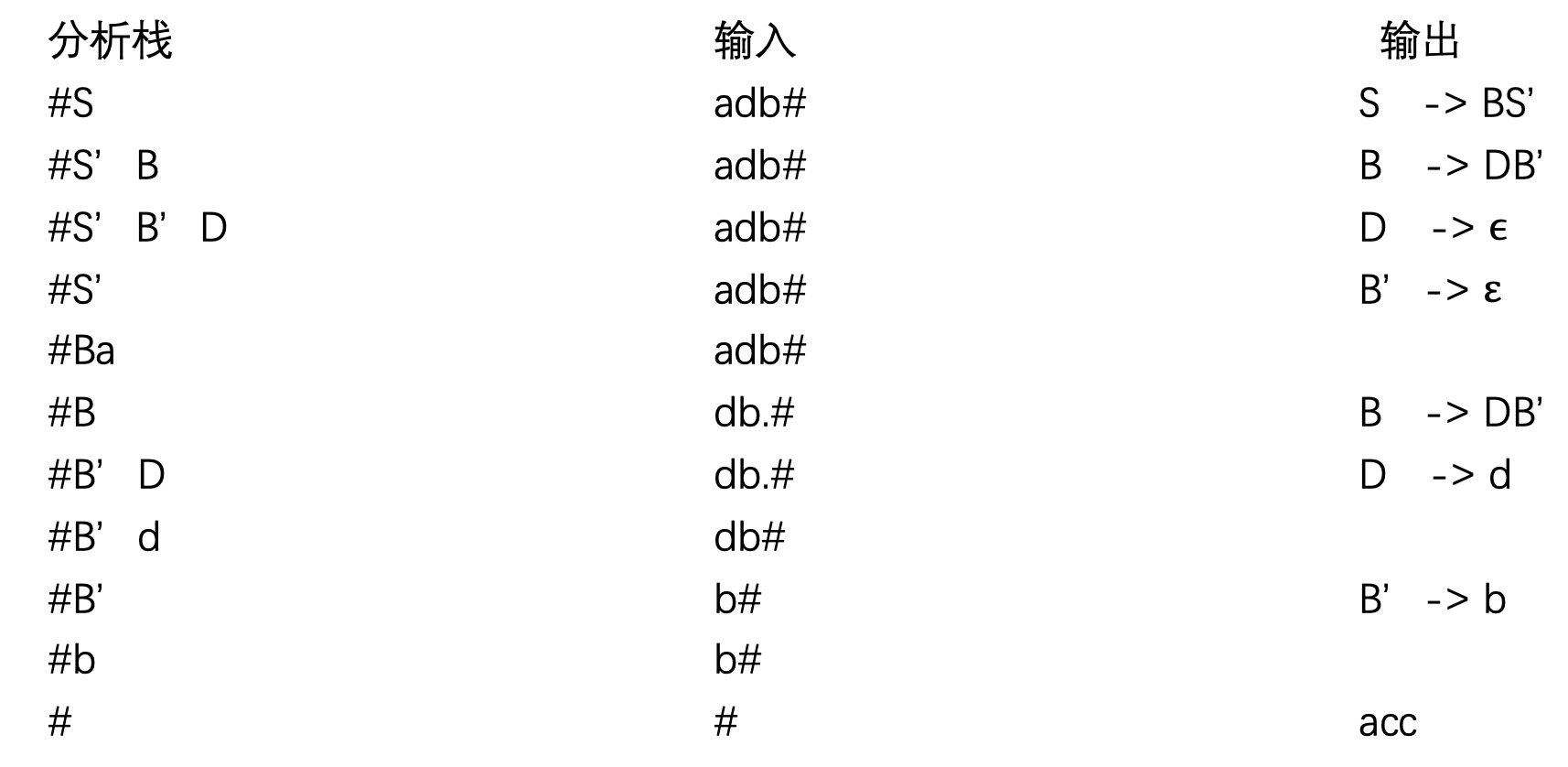解释：
将开始符号S放入栈顶，要分析的句子放入输入，看分析表，当S遇到a时，根据第三条规则，执行 S → \rightarrow BS ‘ ^ ,将S从栈顶逐出，将BS ‘ ^ 按反序推进栈，得到S ‘ ^ B，看分析条，当B遇到a，根据第三条规则，执行 B → \rightarrow DB ‘ ^ , 将B从栈顶逐出，将DB ‘ ^ 按反序推进栈，得到B ‘ ^ D, 看分析表 …(依此类推)，当分析栈为终结符时，则根据第二条规则，分析栈栈顶和输入串第一个字符进行抵消，当分析栈栈顶为‘#’，且输入串为‘#’时，则宣布分析成功，停止分析过程
展开全文编译原理 FIRST集 FOLLOW集
• 编译原理：FIRST集与FOLLOW集

千次阅读 2018-12-13 20:06:28FIRST follow
• 编译原理FIRST集和FOLLOW集的求法

千次阅读 多人点赞 2019-06-18 17:16:41
编译原理FIRST集和FOLLOW的求法 由于最近要期末考试了，所以要从头到尾复习（预习）一遍了。陈意云老师写的课本比较抽象，看不太懂，最后又找了中科大的编译原理课，可能听的时候太困了没有集中精力，又跑去看了...编译原理
• 编译原理 —— FIRST集

千次阅读 2019-02-05 18:24:41
给定一个文法符号串α，α的串首终结符 FIRST（a） 被定义为可以从a推导出的所有串首终结符构成的集合。 如果 α=&amp;amp;amp;amp;amp;amp;amp;amp;gt;∗εα=&amp;amp;amp;amp;amp;amp;amp;amp;gt;^*ε...
• #输入文法求First集和Follow #要求大写字母表示非终结符，小写字母表示终结符 #最后一个产生式以$结尾或者输入$表示输入结束 #默认第一个产生式的→左边为起始符号 def inputGrammer(): #接收文法输入的函数 ...python 编译原理 First集 Follow集
• Vt是终结符，Vn是非终结符，P为产生式，S为开始的非终结符。（书上一般大写的是非终结符，小写是终结符） 以下的定义可直接不看，书上太抽象，又啰嗦，直接看红字...称FIRST(a)为a的开始符号或首符号。 ...编译原理
• 编译原理求FIRST集合FOLLOW集，超简单

千次阅读 多人点赞 2020-12-03 19:16:13
求谁的FIRST集，要找谁在左侧的产生式，拿书上的例子来说（编译原理，陈火旺，第三版） E->TE' E'->+TE'|@ T->FT' T'->*FT'|@ F->(E)|i 然后要看产生式右侧， 1、若产生式右侧以终结符开头，就把这...经验分享
• First集 定义：对于任意文法符号串α ，FIRST(α)是可从α推导得到的串的首符号的集合 如果αε，则ε也在FIRST(α)中（ 即α可空） FIRST(α)={t|α-->tβ, t∈T}U{ε|α-->ε} 做法：首先明确FIRST...
• 编译原理求FIRST集、FOLLOW集和SELECT集

万次阅读 多人点赞 2019-07-02 15:29:50
觉得解释比较不错。 所有大写字母代表非终结符，小写字母代表终结符，省略号代表未知数目（可能为0）的不确定类型的文法符号。 First集合： First集合顾名思义就是求一个文法符号串所...求First集合可分如下几...
• 实验一：状态转换图 输入一串数据，利用状态转换图程序求出“关键字，标识符，整数，运算符，实数”。 实验二：DFA扫描 打开一个编写好的源...输入一个不含左递归的文法，由此程序求出该文法的first集和follow
• 编译原理------C++实现求First集和Follow集

千次阅读 多人点赞 2019-12-06 20:39:54
First集算法描述 1.若X->a…，则将终结符a放入First（X）中 2.若X->ε，则将ε放入First（X）中 3.若有X->Y1Y2Y3…Yk，则 （1）把First（Y1）去掉 ε后加入First（X） （2）如果First（Y1）包含ε，则...编译原理 c++
• 能够根据用户给定的任意...改写文法为等价的LL(1)文法，消除左递归，消除左因子，求每个非终结符的First集合和Follow集合，构造预测分析表,输入测试句式，给出判定结果，并说明判定依据。 参见博客代码介绍，功能全面。
• first集 定义 first(x)：可以从x推导出的所有串首终结符构成的集合 若x→ ε ，ε ∈first(x) follow 定义 follow(A)是可能在某个句型紧跟在A后面终结符a的集合 如果A是某个句型的最右符号，则将终结符\$加入到...
• first集 编译原理 c++版本first集 编译原理 c++版本
• 自上而下分析：FIRST集求法 First集合最终是对产生式右部的字符串而言的，但其关键是求出非终结符的First集合，由于终结符的First集合就是它自己，所以求出非终结符的First集合后，就可很直观地得到每个字符串的...
• 博文链接：https://zpchen.iteye.com/blog/208947
• 编译原理课上实验first集和follow求法：First集合：First集合顾名思义就是求一个文法符号串所可能推导出的符号串的第一个终结符的集合。First（X）就是求X所有推导出的符号串的第一个符号的集合。求First集合可分...编译原理 first集 follow集
• 写了两天的代码才写出来的，本来想多要点分的，想到我去找习题答案的时候花了很大功夫，就算了，跟大家共享，一定给我顶上啊~~~~~ 有陈火旺版的编译原理习题答案和我自己用类C写的编译原理FIRST集求解的源代码，里面......